# Texas Go Math Grade 3 Lesson 18.5 Answer Key Customary Units for Capacity

Refer to our Texas Go Math Grade 3 Answer Key Pdf to score good marks in the exams. Test yourself by practicing the problems from Texas Go Math Grade 3 Lesson 18.5 Answer Key Customary Units for Capacity.

## Texas Go Math Grade 3 Lesson 18.5 Answer Key Customary Units for Capacity

Investigate

Capacity is the amount a container will hold. Customary units used to measure capacity are cup (c), pint (pt), quart (qt), and gallon (gal).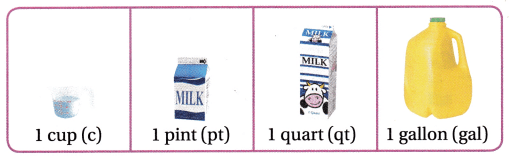Materials; cup, pint, quart, and gallon containers; waterMath Idea
The smallest of these customary units of capacity is a cup.

A. Estimate the number of cups it will take to fill the pint container. Record your estimate.

B. Fill a cup and pour it into the pint container. Repeat until the pint container is full.

C. Record the number of cups it took to fill the pint container.

D. Repeat Steps A to C for the quart and gallon containers.

How many cups are in a pint? _______ in a quart? ________ in a gallon? __________
2 cups in a pint, 4 cups in a quart and 16 cups in a gallon.

Explanation: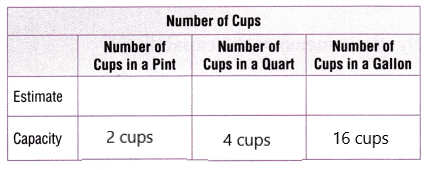Make Connections

These drawings show how cups, pints, quarts, and gallons are related.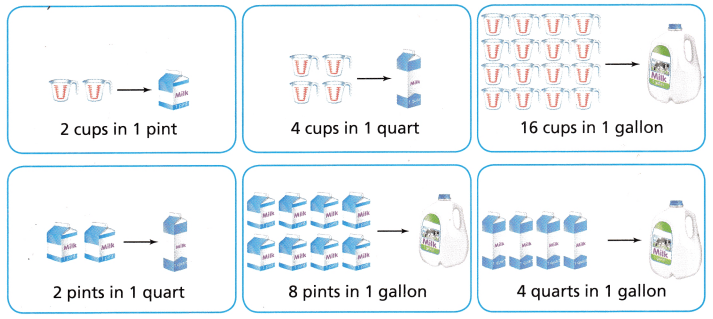There are 2 cups in a pint and 4 cups in a quart. It is double, just like the relationship between cups and pints.
There are 4 cups in a quart and 16 cups in a gallon. It is 4 times as much, just like the connection between quarts and gallons.

Share and Show

Choose the unit you would most likely use to measure the capacity. Write cup, pint, quart, or gallon.

Question 1.cup

Explanation:
The unit that would most likely use to measure the capacity is cup.

Question 2.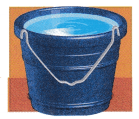Gallon

Explanation:
The unit that would most likely use to measure the capacity is Gallon.

Question 3.Quart or pint

Explanation:

Explanation:
The unit that would most likely use to measure the capacity is Quart or pint.

Question 4.cup or pint

Explanation:
The unit that would most likely use to measure the capacity is cup or pint.

Circle the groups that equal the unit named. Then, rename the capacity using the unit shown.

Question 5.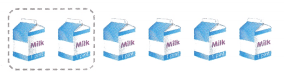6 pints in _________ quarts
3 quarts

Explanation:
6 pints in 3 quarts

Question 6.8 cups in __________ pints
4 pints

Explanation:
8 cups in 4 pints

Problem Solving

Question 7.
Multi-Step Janet is serving lemonade. Each glass contains 1 pint. If Janet serves 16 glasses, how many gallons will she serve?
2 gallons

Explanation:
Janet will serve 2 gallons of lemonade

Question 8.
Write Math What’s the Error? Conner says that it takes 8 cups to fill a 2-gallon container. Is he correct? Explain.
No

Explanation:
From the given question we can tell 32 cups fill 2 gallons, 4 cups fill 1 quart, and 4 quarts fill a gallon, so 4 × 4 = 16 cups fill 1 gallon, and
32 cups fill 2 gallons.

Question 9.
H.O.T. Multi-Step Mr. Velez has 8 pints of orange juice and 1 gallon of grape juice. How many quarts of juice does he have? Explain.8 quarts

Explanation:
2 pints = 1 quart, so 8 pints = 4 quarts. Add the 4 quarts in a gallon. 4 + 4 = 8.

Question 10.
Reasoning What unit of capacity would you use to measure the amount of water in a bathtub?
Gallon

Explanation:
From the given question a bathtub holds a lot of water and a gallon is the largest unit of capacity.

Question 11.
H.O.T. Which is the greater capacity, 2 gallons or 7 quarts? Justify by drawing a picture.
2 gallons

Explanation:

Question 12.
What’s the Question? Angela used the drawing below to help answer a question. The answer is 1 quart.How can you rename 4 cups using 1 quart.

Fill in the bubble for the correct answer choice.

Question 13.
Tiger asks Shakira for 1 quart of milk for his tea. How many pints of milk does Shakira need to give Tiger?
(A) 2 pints
(B) 4 pints
(C) 3 pints
(D) 8 pints
2 pints

Explanation:
Shakira need to give 2 pints of milk.

Question 14.
Steve buys a 2-quart container of orange juice. How many cups of orange juice does he buy?
(A) 3 cups
(B) 8 cups
(C) 4 cups
(D) 2cups
8 cups

Explanation:
He needs to buy 8 cups of orange juice.

Question 15.
Multi-Step Mr. Armstrong is serving 8 quarts of cranberry juice and 3 gallons of apple juice at a party. Each glass contains 2 cups. How many glasses will Mr. Armstrong serve?
(A) 80 glasses
(B) 40 glasses
(C) 16 glasses
(D) 24 glasses
40 glasses

Explanation:
Mr. Armstrong serves 40 glasses of cranberry juice.

Texas Test Prep

Question 16.
Emily enjoys drinking cocoa before she goes to bed at night. Choose the amount that her mug could hold?
(A) 4 quarts
(B) 2 gallons
(C) 2 cups
(D) 4 pints
2 cups

Explanation:
The amount that her mug could hold is 2 cups.

### Texas Go Math Grade 3 Lesson 18.5 Homework and Practice Answer Key

Choose the unit you would use to measure the capacity. Write cup, pint, quart, or gallon.

Question 1.Gallon

Explanation:
Gallon is the unit you would use to measure the capacity.

Question 2.Quart

Explanation:
Quart is the unit you would use to measure the capacity.

Question 3.Cup

Explanation:
Cup is the unit you would use to measure the capacity.

Circle the groups that equal the unit named. Then, rename the capacity using the unit shown.

Question 4.8 quarts in _________ gallons
2 gallons

Explanation:
8 quarts in 2 gallons

Question 5.4 pints in __________ quarts
2 quarts

Explanation:
4 pints in 2 quarts

Question 6.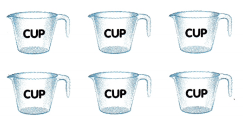6 cups in _________ pints
3 pints

Explanation:
6 cups in 3 pints

Problem Solving

Question 7.
Jacey’s fish tank holds 12 quarts of water. How many gallons of water should Jacey use to fill his fish tank?
3 gallons

Explanation:
Jacey used to fill his fish tank with 3 gallons of water.

Question 8.
Selena has a cooking pot that holds 3 quarts of water. How many cups of water does she need to fill the pot?
12 cups

Explanation:
She need to fill the pot with 12 cups of water.

Lesson Check

Question 9.
Zuri wants to fill a 1-gallon jug with pints of juice. How many pints of juice does Zuri need to fill the jug?
(A) 8 pints
(B) 4 pints
(C) 2 pints
(D) 6 pints
8 pints

Explanation:
Zuri need to fill the jug with 8 pints of juice.

Question 10.
Tanner buys a 1-gallon jug of milk. How many cups of milk can Tanner pour from the gallon?
(A) 10 cups
(B) 8 cups
(C) 16 cups
(D) 12 cups
16 cups

Explanation:
Tanner pour 16 cups of milk from the gallon.

Question 11.
Henley needs 2$$\frac{1}{2}$$ quarts of water to make a batch of chicken soup. How many pints of soup will Henley make?
(A) 4 pints
(B) 3 pints
(C) 5 pints
(D) 6 pints
5 pints

Explanation:
Henley make 5 pints of chicken soup.

Question 12.
Chen places a large pot on the stove to cook spaghetti. Choose the amount that Chen’s pot could hold if it were full.
(A) 8 gallons
(B) 3 quarts
(C) 2 cups
(D) 1 pint
3 quarts

Explanation:
The amount that Chen’s pot could hold it it were full is 3 quarts.

Question 13.
Multi-Step Mr. Tome uses 5 quarts of pineapple juice and 2 gallons of orange juice to make punch. How many 1-cup servings of punch will Mr. Tome make?
(A) 20 cups
(B) 16 cups
(C) 40 cups
(D) 52 cups
52 cups

Explanation:
Mr. Tome makes 52 cups of 1-cup servings of punch.

Question 14.
Multi-Step Garcia has a 5-gallon fish tank and a 3-gallon fish tank. How many more pints of water are in the 5-gallon tank than in the 3-gallon tank?
(A) 16 pints
(B) 40 pints
(C) 24 pints
(D) 30 pints